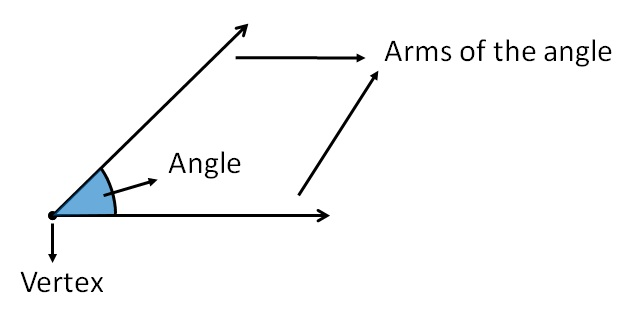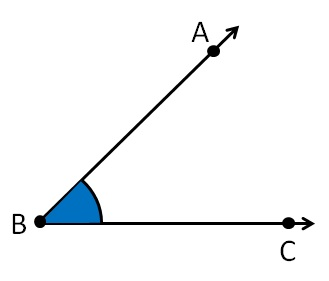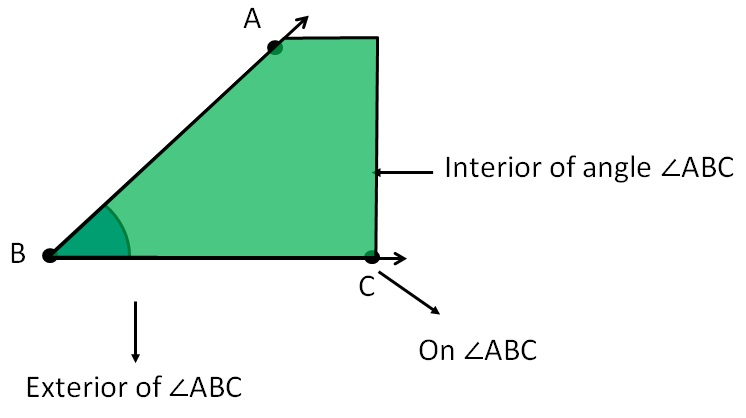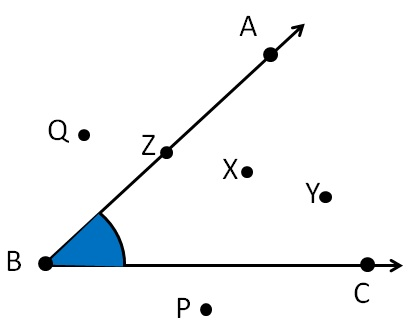Angles

Chapter 4 Class 6 Basic Geometrical Ideas
Concept wiseAngle is formed between two rays with a common end point.The common end point is called vertex of the angle

and the rays are called arms of the angle.

#### How do we name the angle?

Suppose the angle isWe can call it

∠ B

Or

∠ ABC

Symbol of angle → ∠

#### Interior and Exterior of an angle

Let us take the angle#### ExampleX, Y is in interior of ∠ABC

Z is on  ∠ABC

P, Q is in exterior of  ∠ABC

Maths Crash Course - Live lectures + all videos + Real time Doubt solving!